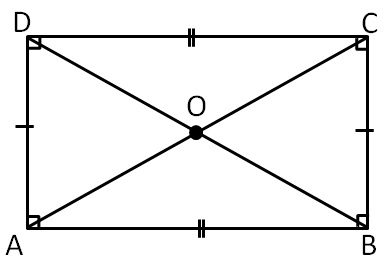Rectangle is a parallelogram where

all angles are 90°

Properties of Rectangle:

Since it is a parallelogram,

it will have all its properties

In parallelogram

• Opposite sides are equal
• Opposite angles are equal
• Diagonals bisect each other
• Sum of adjacent angles is 180°

Other properties are

Diagonals are of equal length

i.e. AC = BD

1. Chapter 4 Class 8 Practical Geometry
2. Concept wise
3. Constructing Rectangle